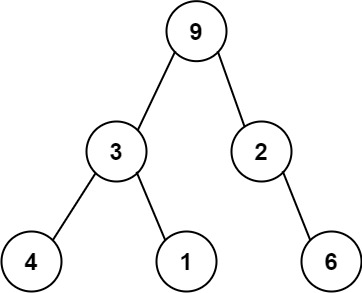# Leetcode 331 - Verify preorder serialization of a binary tree

Note:

• DFS
• Why would it work? If we can finish iterating the preorder array, it means it’s a good binary tree.
• What if there are some extra nodes at the end? Then res !== preorder.length.
• What if there are some nodes missing? Because we check i >= preorder.length, we can still get false.
•• Indegree & Outdegree.
• For a binary tree, indegree === outdegree
• Root node provides 2 outdegree.
• # node provides 1 indegree.
• Normal node provides 1 indegree and 2 outdegree.

Question:

One way to serialize a binary tree is to use preorder traversal. When we encounter a non-null node, we record the node’s value. If it is a null node, we record using a sentinel value such as '#'.For example, the above binary tree can be serialized to the string "9,3,4,#,#,1,#,#,2,#,6,#,#", where '#' represents a null node.

Given a string of comma-separated values preorder, return true if it is a correct preorder traversal serialization of a binary tree.

It is guaranteed that each comma-separated value in the string must be either an integer or a character '#' representing null pointer.

You may assume that the input format is always valid.

• For example, it could never contain two consecutive commas, such as "1,,3".

Note: You are not allowed to reconstruct the tree.
Example:

Code: